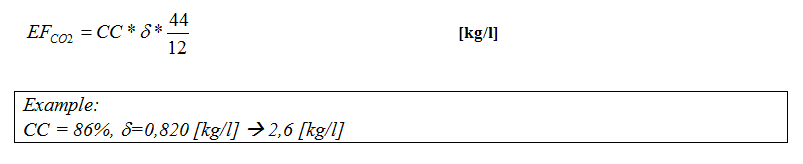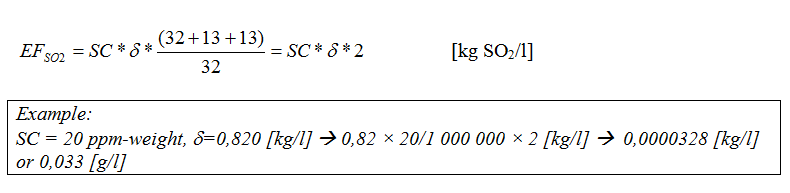# 5.6 Emissions proportional to the fuel consumption

Methods and manuals > 5. Road cargo transport > Wikis > 5.6 Emissions proportional to the fuel consumption
Methods and manuals > 5. Road cargo transport > Wikis > 5.6 Emissions proportional to the fuel consumption

The non-regulated emissions of CO2 and SO2 are calculated based on the physical data for the fuel, i.e. carbon (C) and sulphur (S) content.

CO2

Under the assumption that all carbon is transformed into CO2, the carbon content [in mass-%] is multiplied with the fuel density and the molecular weight relations, (12+16+16)/12=44/12 (molecular weight of CO2 = 44 and Carbon = 12).

Carbon dioxide is calculated by using the following information of the fuel:

carbon content (CC)                            %-weight

density (d)                                           [kg/l]

The emission factor is calculated by applying equation below:Equation for calculating CO2 emission factor

NOTE: This method neglects the fact that a small amount of carbon leaves the engine as CO, hydrocarbons and particles.

SO2

The sulphur in the fuel is oxidised during the combustion process. As a result SO2 is formed. A small amount of sulphur can also be emitted as SO3 or as sulphite och sulphate in the particle phase.

Sulphur dioxide emission is calculated by using the following information of the fuel:

Fuel sulphur content (SC)                   [ppm-weight]

Fuel density (d)                                   [kg/l]

The emission is calculated by applying the equation below:NOTE: This method neglects the fact that a small amount of sulphur and sulphur oxides leaves the engine as condensed droplets and/or attached to particles.